1. /
2. CBSE
3. /
4. Class 07
5. /
6. Mathematics
7. /
8. NCERT Solutions for Class...

# NCERT Solutions for Class 7 Maths Exercise 14.3### myCBSEguide App

Download the app to get CBSE Sample Papers 2023-24, NCERT Solutions (Revised), Most Important Questions, Previous Year Question Bank, Mock Tests, and Detailed Notes.

NCERT solutions for Maths Symmetry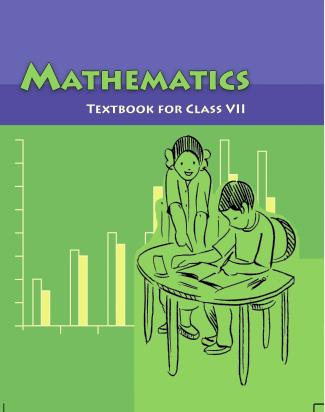## NCERT Solutions for Class 7 Maths Symmetry

###### Question 1. Name any two figures that have both line symmetry and rotational symmetry.

NCERT Solutions for Class 7 Maths Exercise 14.3

###### Question 2. Draw, wherever possible, a rough sketch of:
1. a triangle with both line and rotational symmetries of order more than 1.
2. a triangle with only line symmetry and no rotational symmetry of order more than 1.
3. a quadrilateral with a rotational symmetry of order more than 1 but not a line symmetry.
4. a quadrilateral with line symmetry but not a rotational symmetry of order more than 1.
1. Answer: (i) An equilateral triangle has both line and rotational symmetries of order more than 1.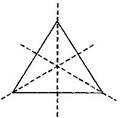Line symmetry:

Rotational symmetry: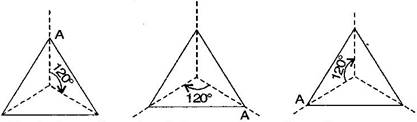(ii)An isosceles triangle has only one line of symmetry and no rotational symmetry of order more than 1.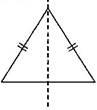Line symmetry: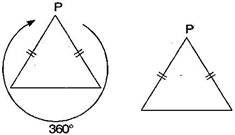Rotational symmetry:

(iii)It is not possible because order of rotational symmetry is more than 1 of a figure, most acertain the line of symmetry.

(iv)A trapezium which has equal non-parallel sides, a quadrilateral with line symmetry but not a rotational symmetry of order more than 1.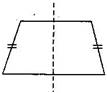Line symmetry:

Rotational symmetry: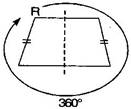NCERT Solutions for Class 7 Maths Exercise 14.3

###### Question 3. In a figure has two or more lines of symmetry, should it have rotational symmetry of order more than 1?

Answer: Yes, because every line through the centre forms a line of symmetry and it has rotational symmetry around the centre for every angle.

NCERT Solutions for Class 7 Maths Exercise 14.3

###### Question 4. Fill in the blanks:
 Shape Centre of Rotation Order of Rotation Angle of Rotation Square Rectangle Rhombus Equilateral triangle Regular hexagon Circle Semi-circle

 Shape Centre of Rotation Order of Rotation Angle of Rotation Square Intersecting point of diagonals. 4 {tex}{90^ \circ }{/tex} Rectangle Intersecting point of diagonals. 2 {tex}{180^ \circ }{/tex} Rhombus Intersecting point of diagonals. 2 {tex}{180^ \circ }{/tex} Equilateral triangle Intersecting point of medians. 3 {tex}{120^ \circ }{/tex} Regular hexagon Intersecting point of diagonals. 6 {tex}{60^ \circ }{/tex} Circle Centre infinite At every point Semi-circle Mid-point of diameter 1 {tex}{360^ \circ }{/tex}

NCERT Solutions for Class 7 Maths Exercise 14.3

###### Question 5. Name the quadrilateral which has both line and rotational symmetry of order more than 1.

Answer: Square has both line and rotational symmetry of order more than 1.

Line symmetry: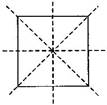Rotational symmetry: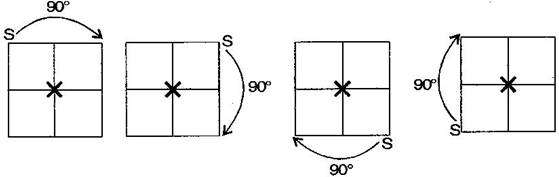NCERT Solutions for Class 7 Maths Exercise 14.3

###### Question 6. After rotating by {tex}{60^ \circ }{/tex} about a centre, a figure looks exactly the same as its original position. At what other angles will this happen for the figure?

Answer: Other angles will be {tex}120^\circ ,180^\circ ,240^\circ ,300^\circ ,360^\circ {/tex}.

For rotation:It will rotate six times.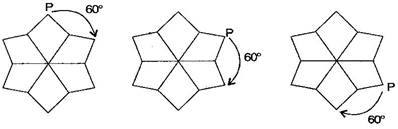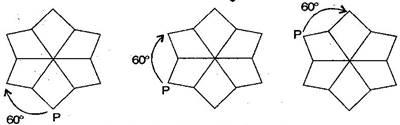For rotation:It will rotate three times.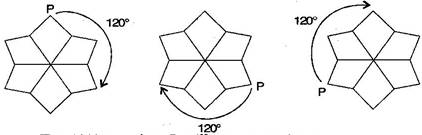For rotation:It will rotate two times.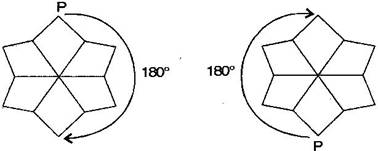For rotation:It will rotate one time.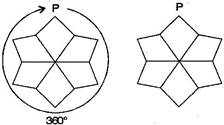NCERT Solutions for Class 7 Maths Exercise 14.3

###### Question 7. Can we have a rotational symmetry of order more than 1 whose angle of rotation is:

(i) {tex}{45^ \circ }{/tex}(ii) {tex}{17^ \circ }{/tex} ?

Answer: (i) If the angle of rotation is {tex}{45^ \circ },{/tex} then symmetry of order is possible and would be 8 rotations.

(ii) If the angle of rotational is {tex}{17^ \circ },{/tex} then symmetry of order is not possible because {tex}{360^ \circ }{/tex} is not complete divided by {tex}{17^ \circ }.{/tex}

## NCERT Solutions for Class 7 Maths Exercise 14.3

NCERT Solutions Class 7 Maths PDF (Download) Free from myCBSEguide app and myCBSEguide website. Ncert solution class 7 Maths includes text book solutions from Class 7 Maths Book . NCERT Solutions for CBSE Class 7 Maths have total 15 chapters. 7 Maths NCERT Solutions in PDF for free Download on our website. Ncert Maths class 7 solutions PDF and Maths ncert class 7 PDF solutions with latest modifications and as per the latest CBSE syllabus are only available in myCBSEguide.

## CBSE app for Students

To download NCERT Solutions for Class 7 Maths, Social Science Computer Science, Home Science, Hindi English, Maths Science do check myCBSEguide app or website. myCBSEguide provides sample papers with solution, test papers for chapter-wise practice, NCERT solutions, NCERT Exemplar solutions, quick revision notes for ready reference, CBSE guess papers and CBSE important question papers. Sample Paper all are made available through the best app for CBSE students and myCBSEguide website.Test Generator

Create question paper PDF and online tests with your own name & logo in minutes.myCBSEguide

Question Bank, Mock Tests, Exam Papers, NCERT Solutions, Sample Papers, Notes

### 3 thoughts on “NCERT Solutions for Class 7 Maths Exercise 14.3”

1. Centre of rotation should be in number

2. Great it is true

3. It is true